# Binary Addition (How To Guide With Rules And Examples)

## How to Add Binary Numbers

We have discussed the topic binary arithmetic as a whole, now we will concentrate on the topic binary addition separately.

Binary addition, binary subtraction, binary multiplication and binary division are the four types of arithmetic operations that occur in the binary arithmetics.

The first and perhaps the most important of them all is binary addition (this also happens to be the easiest of them all).

When adding binary numbers, there are four points or steps to remember before proceeding through the operation. These are: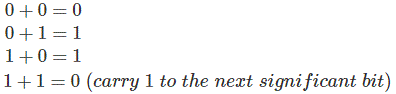Is there no numbers other than 0 and 1 in the binary number system these four steps include all the possible operations of addition.

Now let us explain the operation through an example

Suppose A = 10010101 and B = 10000110

Now we want to find out A + B i.e. we have to perform binary addition, we will look through the process with a thorough explanation.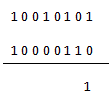This is the addition of the first two least significant digits which are 0 and 1 and 1 + 0 = 1 which is written.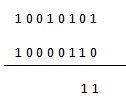Here again the similar step is done i.e. 0 + 1 = 1.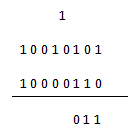Here 1 + 1 = 0 and the carry is written on the top of the next significant bit which will be used in the next step.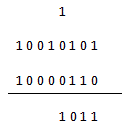As we know 0 + 0 = 0 and 1 + 0 = 1 (1 comes from the carry) and the result 1 is written.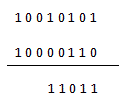Again 1 + 0 = 1 and that is exactly what is written.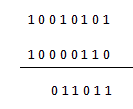The next two bits which are to beaded are 0 and 0 and 0 + 0 = 0.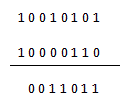Again the same operation 0 + 0 = 0 is done and written.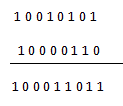The last two bits include 1 + 1 = 0 and carry 1 which is written directly in the result.

The use of binary addition in the digital electronics field can be seen clearly, we have discussed the logic gates which are perhaps the most important part of digital electronics are nothing but the binary arithmetic operations, the XOR gate is the pure binary addition.

Want To Learn Faster? 🎓
Get electrical articles delivered to your inbox every week.
No credit card required—it’s 100% free.